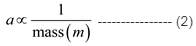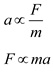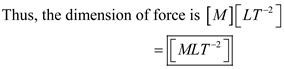# Newton’s second law states that acceleration is proportional to the force

Newton’s second law (to be discussed in Chapter 5) states that acceleration is proportional to the force acting on an object and is inversely proportional to the object’s mass. What are the dimensions of force?